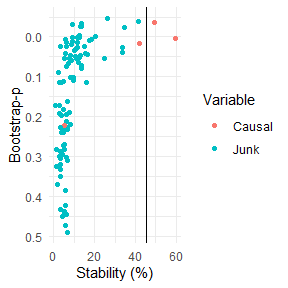# stabiliser

The goal of the stabiliser package is to provide a flexible method of applying stability selection (Meinshausen and Buhlmann, 2010) with various model types, and the framework for triangulating the results for multiple models (Lima et al., 2021).

• stabilise() performs stability selection on a range of models to identify causal models.
• triangulate() identifies which variables are most likely to be causal across all models.
• stab_plot() allows visualisation of either stabilise() or triangulate() outputs.

## Installation

You can install this package from github using the devtools package as follows:

devtools::install_github("roberthyde/stabiliser")

## Usage

The stabiliser_example dataset is a simulated example with the following properties:

• 1 simulated outcome variable: y
• 4 variables simulated to be associated with y: causal1, causal2
• 95 variables simulated to have no association with y: junk1, junk2
library(stabiliser)
data("stabiliser_example")

### stabilise()

To attempt to identify which variables are truly “causal” in this dataset using a selection stability approach, use the stabilise() function as follows:

set.seed(8141)
stable_enet <- stabilise(data = stabiliser_example,
outcome = "y")

Access the stability (percentage of 100 bootstrap resamples where a given variable was selected by a given model) results for elastic net as follows:

stable_enet$enet$stability
#> # A tibble: 100 x 7
#>    variable mean_coefficient ci_lower ci_upper bootstrap_p stability stable
#>    <chr>               <dbl>    <dbl>    <dbl>       <dbl>     <dbl> <chr>
#>  1 causal1              2.82   0.185      6.10           0      85   *
#>  2 causal2              2.17   0.0609     5.31           0      83.5 *
#>  3 causal3              2.82   0.249      6.33           0      71   <NA>
#>  4 junk86               1.51   0.139      4.16           0      69   <NA>
#>  5 junk14               2.71   0.174      6.64           0      65   <NA>
#>  6 junk13               2.14   0.106      5.23           0      63.5 <NA>
#>  7 junk45               2.43   0.127      6.89           0      61.5 <NA>
#>  8 junk88               2.23   0.142      4.78           0      61   <NA>
#>  9 junk90               1.74   0.0429     5.11           0      50.5 <NA>
#> 10 junk26               1.51   0.0417     4.04           0      45.5 <NA>
#> # ... with 90 more rows

This ranks the variables by stability, and displays the mean coefficients, 95% confidence interval and bootstrap p-value. It also displays whether the variable is deemed “stable”, in this case 2 out of the 4 truly causal variables are identified as “stable”, with no false positives.

By default, this implements an elastic net algorithm over 100 bootstrap resamples of the dataset. Stability of each variable is then calculated as the proportion of bootstrap repeats where that variable is selected in the model.

stabilise() also permutes the outcome several times (5 by default) and performs the same process on each permuted dataset (20 bootstrap resamples for each by default).

This allows a permutation threshold to be calculated. Variables with a non-permuted stability % above this threshold are deemed “stable” as they were selected in a higher proportion of bootstrap resamples than in the permuted datasets, where we know there is no association between variables and the outcome.

The permutation threshold is available as follows:

stable_enet$enet$perm_thresh
#>  78.5

### triangulate()

Our confidence that a given variable is truly associated with a given outcome might be increased if it is identified in multiple model types.

Specify multiple model types (elastic net, mbic and mcp) for comparison using the stabilise() function as follows:

set.seed(8141)
stable_combi <- stabilise(data = stabiliser_example,
outcome = "y",
models = c("enet",
"mbic",
"mcp"))

The stability of variables is available for all model types as before.

The stability results from these three models stored within stable_combi can be combined using triangulate as follows:

triangulated <- triangulate(stable_combi)
triangulated
#> $combi #>$combi$stability #> # A tibble: 100 x 4 #> variable stability bootstrap_p stable #> <chr> <dbl> <dbl> <chr> #> 1 causal1 58.5 0 * #> 2 causal2 48.7 0 * #> 3 causal3 41.8 0 <NA> #> 4 junk88 40.7 0.00444 <NA> #> 5 junk14 35 0 <NA> #> 6 junk13 34.7 0 <NA> #> 7 junk86 33.2 0 <NA> #> 8 junk45 26.5 0 <NA> #> 9 junk90 24.3 0.0101 <NA> #> 10 junk26 20 0.0128 <NA> #> # ... with 90 more rows #> #>$combi\$perm_thresh
#>  45.33333

This shows variables consistently being identified as being stable across multiple model types, and consequently increasing our confidence that they are truly associated with the outcome.

Triangulating the results for stability selection across multiple model types generally provides better performance at identifying truly causal variables than single model approaches (Lima et al., 2021). As shown in this example, the triangulated approach identifies 3 out of the 4 truly causal variables as being “stable”, and being highly likely to be associated with the outcome y, in contrast to the 2 variables identified when using elastic net alone.

### stab_plot()

Both stabilise() and triangulate() outputs can be plotted using stab_plot() as follows, with causal and junk variables highlighted for this example:

stab_plot(stabiliser_object = triangulated)